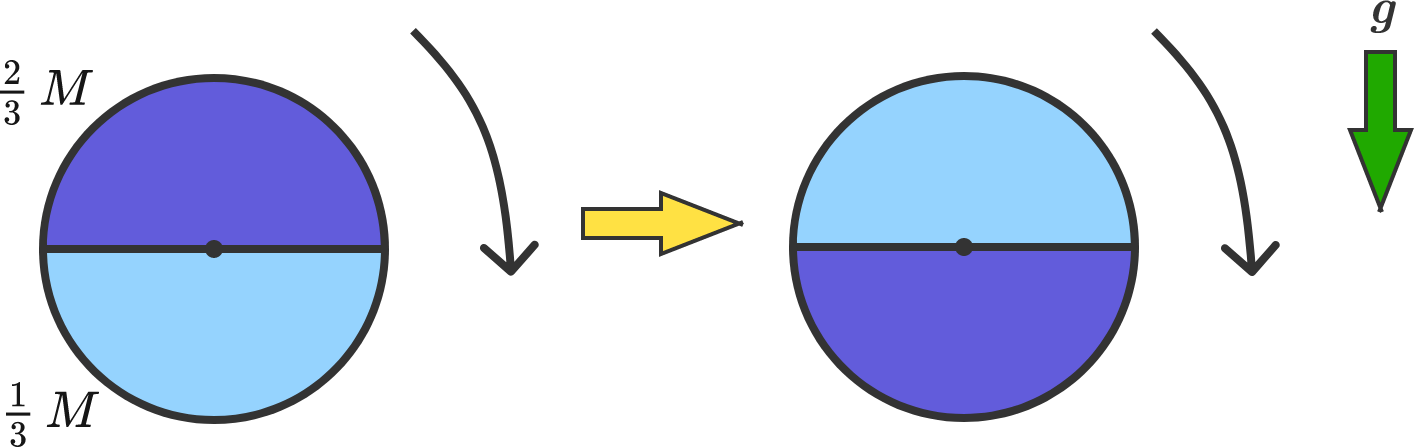# Uneven Disk Toppling OverA circular disk of radius $R$ consists of two uniform halves of equal area with masses $\frac{2}{3}M$ and $\frac{1}{3}M$. The disk is free to rotate on an axle through its geometric center.

Initially, the disk is at rest as shown on the left such that the half of mass $\frac23 M$ is on top.

Suppose the disk is toppled by an extremely gentle nudge. At the instant the disk first reaches the orientation on the right, its angular speed can be expressed as follows:

$\omega = \sqrt{\frac{Ag}{B \pi R}}.$

If $A$ and $B$ are coprime positive integers, determine $A + B$.

×